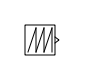# Repeating Sequence

Generate arbitrarily shaped periodic signal

•Libraries:

## Description

The Repeating Sequence block outputs a periodic scalar signal having a waveform that you specify using the Time values and Output values parameters. The Time values parameter specifies a vector of output times. The Output values parameter specifies a vector of signal amplitudes at the corresponding output times. Together, the two parameters specify a sampling of the output waveform at points measured from the beginning of the interval over which the waveform repeats (the period of the signal).

By default, both parameters are `[0 2]`. These default settings specify a sawtooth waveform that repeats every `2` seconds from the start of the simulation and has a maximum amplitude of `2`.

## Ports

### Output

expand all

Output signal specified by the Time values and Output values parameters to create a periodic scalar signal.

Data Types: `double`

## Parameters

expand all

Vector of strictly monotonically increasing time values. The period of the generated waveform is the difference of the last and first values of this parameter.

#### Programmatic Use

 Block Parameter: `rep_seq_t` Type: character vector Values: vector Default: ```[0 2]```

Vector of output values that specify the output waveform. Each element corresponds to the time value in the Time valuesparameter.

#### Programmatic Use

 Block Parameter: `rep_seq_y` Type: character vector Values: vector Default: ```[0 2]```

## Block Characteristics

 Data Types `double` Direct Feedthrough `no` Multidimensional Signals `no` Variable-Size Signals `no` Zero-Crossing Detection `no`

## Algorithms

The block sets the input period as the difference between the first and last value of the Time values parameter. The output at any time `t` is the output at time `t = t-n*period`, where `n` is an integer. The sequence repeats at ```t = n*period```. The block uses linear interpolation to compute the value of the waveform between the output times that you specify.

## Version History

Introduced before R2006a